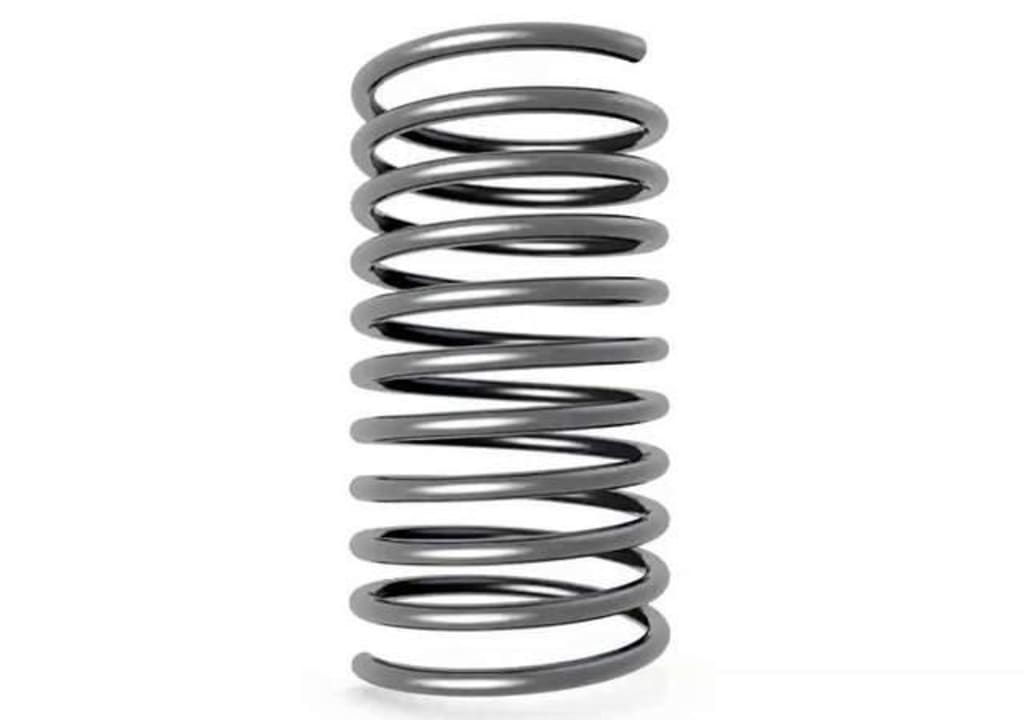# Put the compressed spring into strong acid

## After the spring dissolves, where does the energy of the spring go?

By ApostolakisPublished 8 months ago 5 min read
LikeSpring

The spring itself is elastic, after being compressed, the spring will have the tendency to return to its initial state, and therefore has elastic potential energy, which we can see as the energy stored in the spring as a whole system, so the question arises: if the compressed spring is put into a strong acid, then after the spring dissolves, where does the energy of the spring go?

The law of conservation of energy tells us that energy cannot disappear out of thin air, and the energy of a spring is certainly no exception.

On a microscopic level, an iron spring is made of a large number of iron atoms combined by inter atomic interactions. Since the nucleus is positively charged and the electrons are negatively charged, the nucleus is attracted to the neighboring electron groups between neighboring atoms, and at the same time, the neighboring nucleus and the nucleus, and the electrons and electrons are repelled by each other.

In other words, the interactions between atoms have both attractive and repulsive forces, the strength of which is closely related to the distance between atoms (as shown in the figure below).

As can be seen, when the distance is smaller than "r", the interaction between atoms is characterized by repulsive forces, when the distance is larger than "r", the interaction between atoms is characterized by attractive forces, and if the distance exceeds a certain level, the interaction between atoms is negligible. If the distance exceeds a certain level, the interaction between atoms is negligible.

When the distance between atoms is "r", the combined force is zero, and only in this equilibrium position can the neighboring atoms remain stable, so when a large number of atoms are combined, they always tend to be in an equilibrium position between atoms.

The picture above shows the basic unit of the crystal structure of iron at room temperature, which is a body-centered cubic lattice in which all the iron atoms vibrate in equilibrium positions between the atoms, and the same is true for the crystal structure inside an uncompressed iron spring.

When the spring is compressed, a large number of lattices are deformed by the compression, which means that a large number of iron atoms deviate from their original equilibrium positions.

In this case, these iron atoms tend to return to their equilibrium position due to the interaction between the atoms, and therefore have potential energy, which is in essence electrical potential energy, which, when superimposed, is expressed on a macroscopic level as the spring's elastic potential energy.

When the spring is dissolved by a strong acid, there is a process in which the iron atoms break away from the main body. For iron atoms that have electrical potential energy, when they break away from the main body, the electrical potential energy is converted into their kinetic energy, in other words, the speed of these iron atoms is faster than the normal state (i.e., the spring is not compressed).

After this, the kinetic energy of these iron atoms is transferred to the other microscopic particles in the strong acid solution in the form of collisions, which eventually manifests itself as heat energy of the strong acid solution, why heat energy? Because at the microscopic level, the essence of heat energy is the thermal motion of microscopic particles.

So we can conclude that if we put a compressed spring into a strong acid and dissolve it, the energy of the spring (elastic potential energy) will be "converted into zero" and then into the heat energy of the strong acid solution, which does not violate the law of conservation of energy.

In other words, all other things being equal, the heat energy released by the compressed spring after it is dissolved by the strong acid is a little more than that of the uncompressed spring, thus making the temperature of the strong acid solution higher. However, this temperature difference is so subtle that we would have to have very sophisticated testing instruments to detect it, and to illustrate this point, let's look at a specific example.

The elastic potential energy can be calculated by the formula "U = 0.5kx^2", where k is the coefficient of elasticity and x is the form variable. Suppose there is a spring with a coefficient of elasticity of 1000 N/m, which can be compressed by at most 0.1 m. Then after compressing this spring, its elastic potential energy will be at most 5 joules, and such a small amount of energy This small amount of energy can only raise the temperature of 1 liter of water by 0.0012 K, which is negligible.

It should be noted that only in the ideal case a spring placed in a strong acid solution would dissolve uniformly, but since the spring's constituent material cannot be completely uniform, and since the stresses after the spring are compressed cannot be completely uniformly distributed, the actual situation should be that each part of the spring dissolves faster or slower.

In this case, the part of the spring that dissolves first will provide some degree of bounce space for the part that dissolves later, and the bounce of the spring will stir up the nearby solution, and in this process, part of the elastic potential energy of the compressed spring will be converted into the kinetic energy of the spring and the kinetic energy of the solution stirred up by the spring.

Science
Like

### Apostolakis

To make scientific， diligence is the mother of success

How does it work?

There are no comments for this story

Be the first to respond and start the conversation.